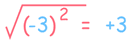# 负数的指数

## 平方消灭负数

• 正数的平方是数： (+5) × (+5) = +25
• 负数的平方也是数： (−5) × (−5) = +25"有啥了不起？"，你说。。。。。。

。。。。。。来看看这个：√(x2) = |x|

## 负数的偶数指数

 1 （奇数）： (-1)1 = -1 2 （偶数）： (-1)2 = (-1) × (-1) = +1 3 （奇数）： (-1)3 = (-1) × (-1) × (-1) = -1 4 （偶数）： (-1)4 = (-1) × (-1) × (-1) × (-1) = +1

(-1)奇数 = -1

(-1)偶数 = +1

97 是奇数，所以：

(-1)97 = -1

26 = 64，6 偶数，所以：

(-2)6 = +64

x2 = -1

x=1 ？

1 × 1 = +1

x=-1 ？

(-1) × (-1) = +1

√-1 不是实数